# E 9 Currents Create Magnetic Fields A moving

• Slides: 13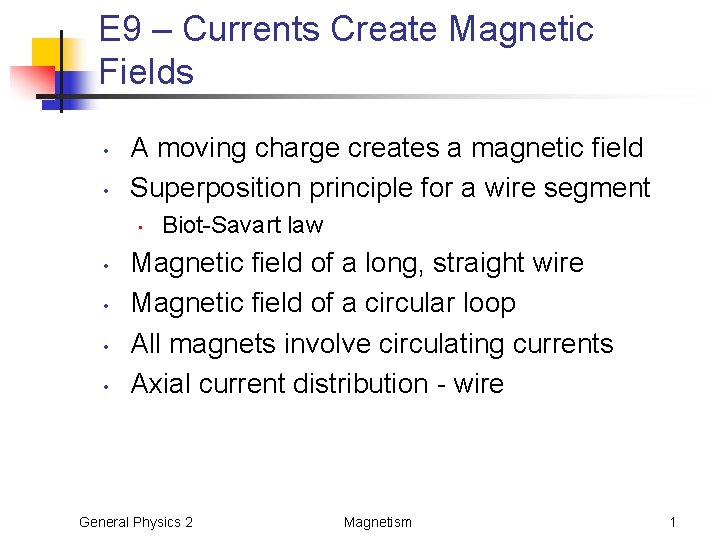E 9 – Currents Create Magnetic Fields • • A moving charge creates a magnetic field Superposition principle for a wire segment • • • Biot-Savart law Magnetic field of a long, straight wire Magnetic field of a circular loop All magnets involve circulating currents Axial current distribution - wire General Physics 2 Magnetism 1Biot-Savart Law • Superposition principle for a moving charge • Superposition principle for wire segments General Physics 2 Magnetism 2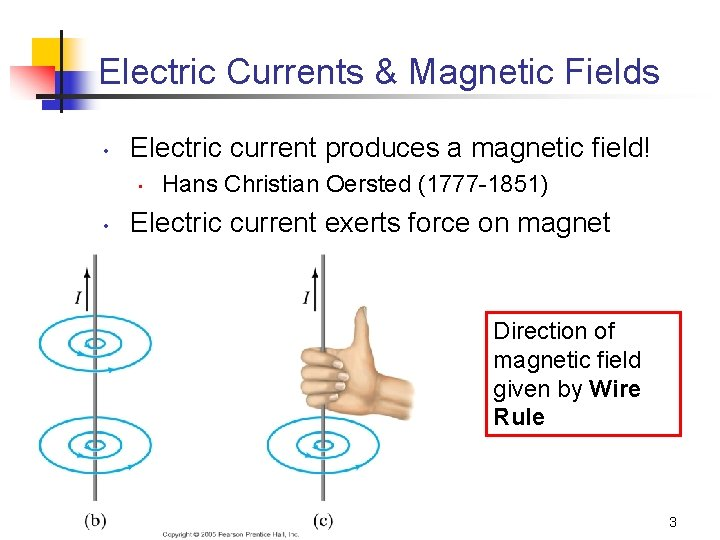Electric Currents & Magnetic Fields • Electric current produces a magnetic field! • • Hans Christian Oersted (1777 -1851) Electric current exerts force on magnet Direction of magnetic field given by Wire Rule General Physics 2 Magnetism 3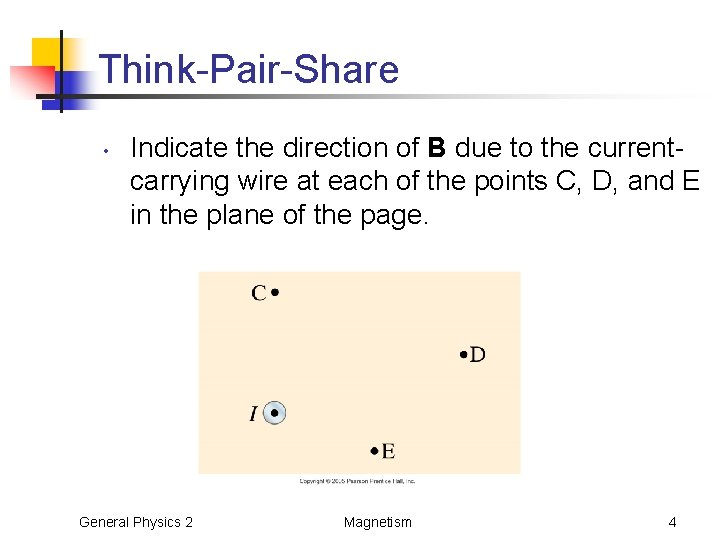Think-Pair-Share • Indicate the direction of B due to the currentcarrying wire at each of the points C, D, and E in the plane of the page. General Physics 2 Magnetism 4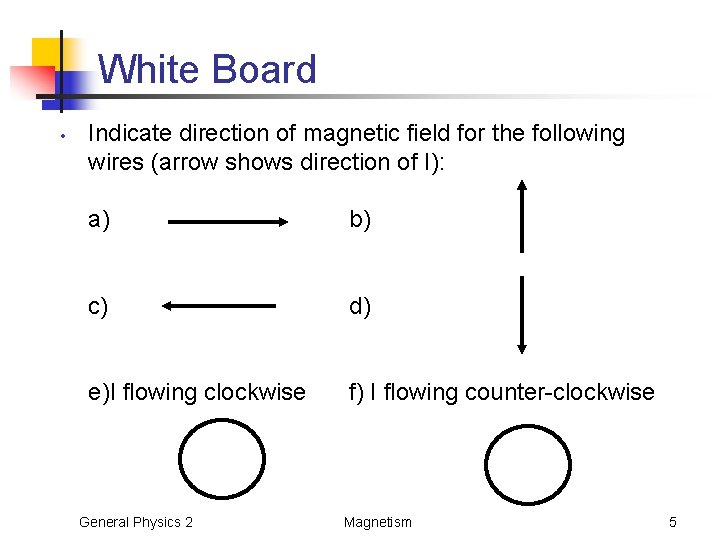White Board • Indicate direction of magnetic field for the following wires (arrow shows direction of I): a) b) c) d) e)I flowing clockwise f) I flowing counter-clockwise General Physics 2 Magnetism 5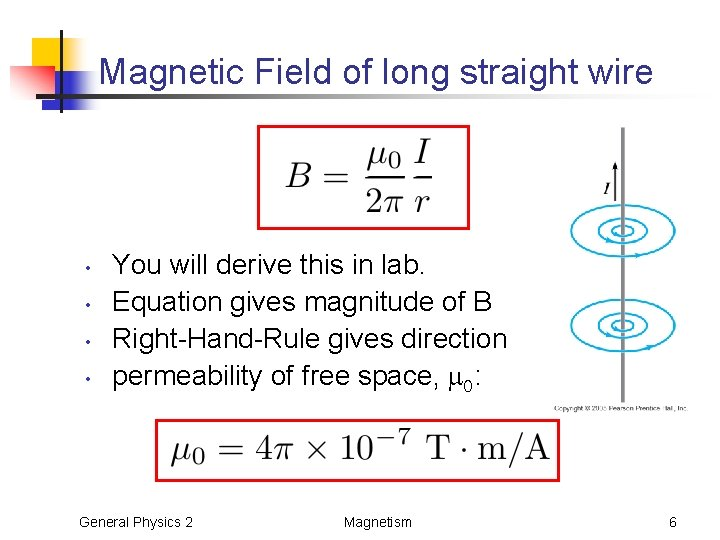Magnetic Field of long straight wire • • You will derive this in lab. Equation gives magnitude of B Right-Hand-Rule gives direction permeability of free space, : General Physics 2 Magnetism 6Magnetic Field of a Current Loop Units of Magnetic Field • Tesla (SI) • 1 T = 1 N/(A m) • Gauss (cgs) • 1 G = 10 -4 T • Earth’s B field: • B = 0. 5 G • B = 0. 5 x 10 -4 T Direction of magnetic field given by Loop Rule General Physics 2 Magnetism 7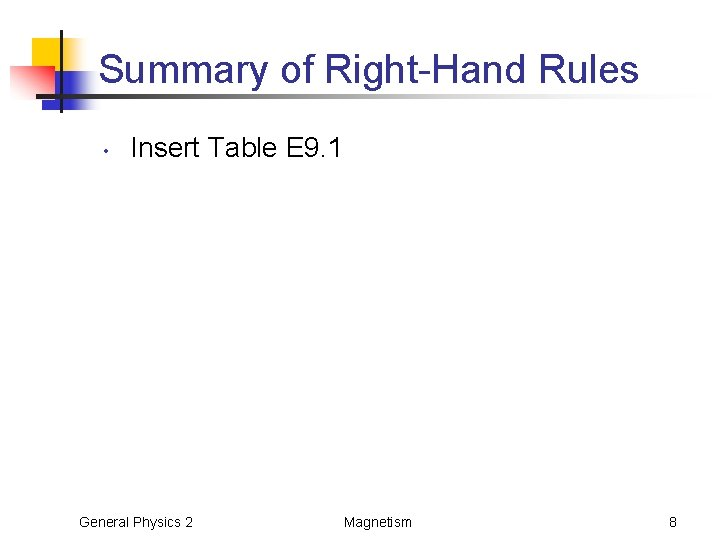Summary of Right-Hand Rules • Insert Table E 9. 1 General Physics 2 Magnetism 8Think-Pair-Share • Two long wires are oriented so that they are perpendicular to each other. At their closest, they are 20. 0 -cm apart. What is the magnitude of the magnetic field at a point midway between them? General Physics 2 Magnetism 9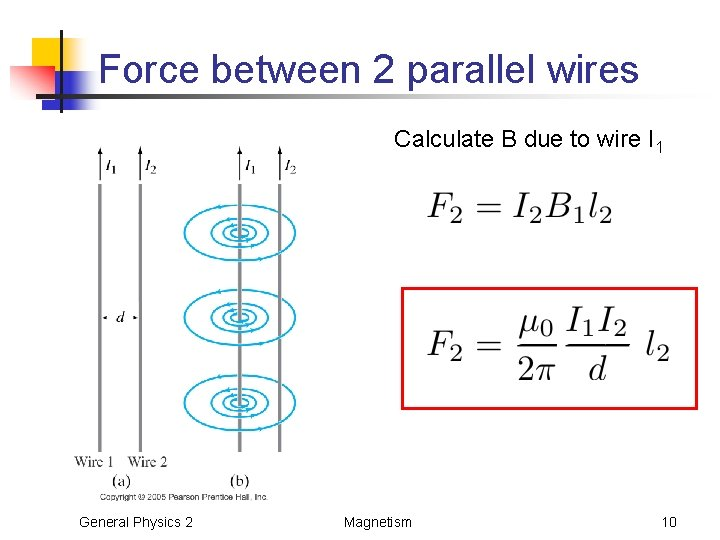Force between 2 parallel wires Calculate B due to wire I 1 General Physics 2 Magnetism 10Find direction of force from Right-Hand-Rule General Physics 2 Magnetism 11Think-Pair-Share • Two long straight parallel wires are 15 cm apart. Wire A carries a 2. 0 A current. Wire B’s current is 4. 0 A in the same direction. (d) Determine the force per length on wire A due to wire B, and the force on wire B due to wire A. General Physics 2 Magnetism 12Practice Problems • Magnetism: • • • Exploration in Physics • • Worksheet 1 Worksheet 3 Magnetism –activities in the hints tab Group Problems • E 9 B. 5, E 9 B. 6, E 9 S. 8 General Physics 2 Magnetism 13